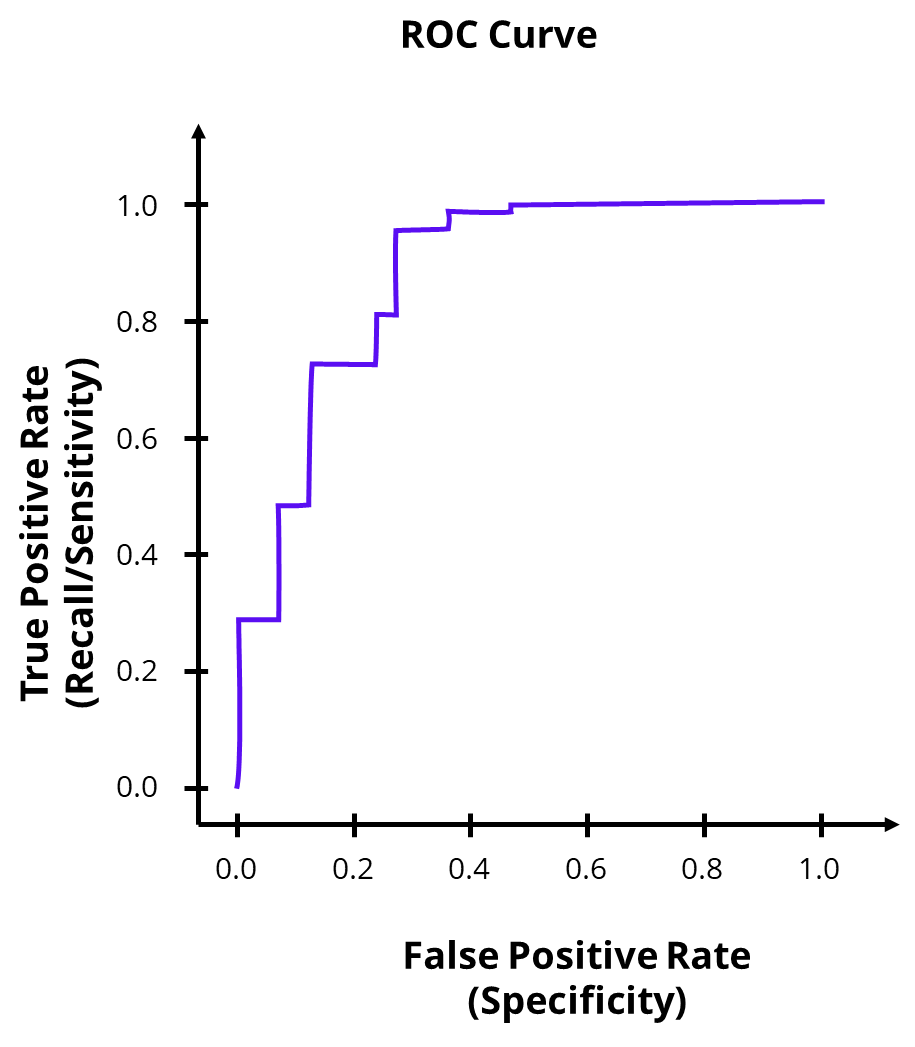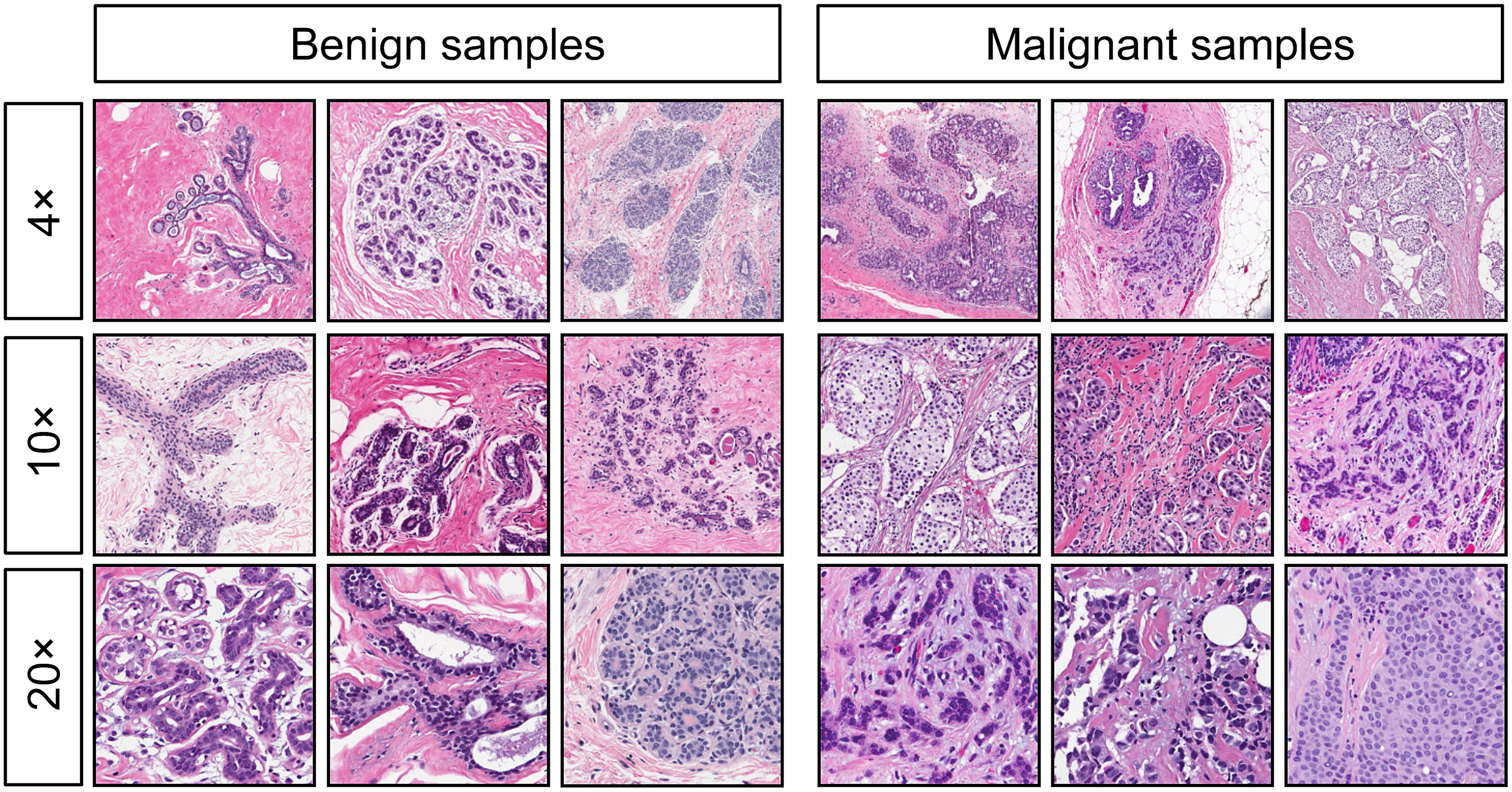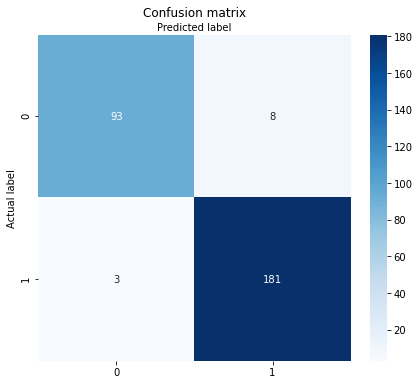# Measuring Classification Performance with Python and Scikit-Learn

Classification is a supervised machine learning problem in which the task is to predict the correct class labels (two or more) for a set of observations. An essential step in developing a classifier is to evaluate its performance. Only when we understand how well a model sorts observations into the correct classes can we build the confidence necessary to use it in production. However, objective evaluation is often tricky because various factors come into play—for example, the distribution and importance of classes. Therefore, a systematic approach to measuring performance relies on proven techniques such as the confusion matrix, error metrics, and the ROC curve. This article provides an overview of these tools and metrics and discusses their potential pitfalls. In addition, we create a simple classifier and apply various techniques to measure its performance using Python and Scikit-Learn.

The rest of this tutorial proceeds in two parts: The first part is conceptual and introduces the essential tools and techniques for evaluating classification performance. The second part of the tutorial is hands-on. We use Python and Scikit-Learn to build a breast cancer detection model classifying tissue samples as benign or malignant. We then apply various techniques to evaluate the model’s performance.

## Techniques for Measuring Classification Performance

This first part of the tutorial presents essential techniques for measuring the performance of classification models, including confusion matrix, error metrics, and roc curves. But why are there so many different techniques? Isn’t it enough to calculate the rate between correct and false classifications?

The answer depends on the balance of the class labels and their importance. Let’s compare a simple two-class case vs. a more complex one. In the most simple case, the following applies:

• The class labels in the sample are perfectly balanced (for example, 50 positives and 50 negatives).
• Both class labels are equally important, so it does not matter if the model is better at predicting class one or two.

In this case, we can measure the model performance as the rate between correctly predicted labels and those that a model falsely predicted. It is as simple as that. However, most classification problems are more complex:

• The class labels are imbalanced, so the model encounters one class more often than the other.
• One class is more important than the other. For example, consider a binary classification problem in which the goal is to identify the few positive cases from a sample with many negative ones. Especially in disease detection, it is crucial that the model correctly identifies the few positive cases, even if some of the observations classified as positive are actually negative.

Confusion matrix and error techniques help us objectively evaluate such models built for more complex problems.

### The Confusion Matrix

A confusion matrix is an essential tool for evaluating a classification model. For a problem where the output may include two classes (negative and positive), the confusion matrix is a table with four different combinations of predicted and actual values. As a result, each prediction falls into one of the following four squares:

• True Positives (TP): the outcome from a prediction is “positive,” and the actual value is also “positive.”
• False Positives (FP): The model predicted a positive value, but this prediction is false.
• True Negatives (TN): Predicted was a negative value, which is correct.
• False Negatives (FN): The model predicted a negative value while the actual class was positive.

We can assign each classification to a cell in the matrix. The diagonal contains the correctly classified cases whose actual class matches the predicted class. All other cells outside the diagonal represent the possible errors. Using the confusion matrix, you can see at a glance how well the model works and what errors it makes.

The confusion matrix is the basis for calculating various error metrics, which we will look at in more detail in the following section.

### Metrics for Measuring Classification Errors

To objectively measure the performance of a classifier, we can count up the cases in the different squares and use this information to calculate essential error metrics, including accuracy, precision, recall, f-1 score, and specificity.

#### Precision

Precision is a metric for the rate of missed positive values. Mathematically, it is the sum of true positives divided by the sum of False Positives and True Positives.

In other words, it measures the ability of a classification model to identify the relevant data points without misclassifying too many irrelevant cases.

$Precision = {TP \over FP + TP}$

#### Accuracy

Accuracy tells us the rate of the positive values that were classified correctly. It is calculated as the sum of all correct classifications divided by the number of false positives.

The usefulness of Accuracy ends when the class labels are imbalanced so that one class is underrepresented. The Accuracy can be misleading as it can become nearly 100% even if the classification model has not identified any of the data points in the underrepresented class. If your data is imbalanced, you should combine accuracy with the Recall.

$Accuracy= {TP + TN \over TP + FN + FP + TN}$
$= {Correct Classifications \over Total Sample Size}$

#### F1-Score

The F1-Score combines the Precision and Recall into a single metric. It is calculated as the harmonic mean of Precision and Recall.

The F1-Score is a single overall metric based on precision and recall. We can use this metric to compare the performance of two classifiers that have different recall and precision.

$F1Score = {TP + TN \over FN}$
$= {2 * Precision * Recall\over Precision + Recall}$

#### Recall (Sensitivity)

Recall, sometimes referred to as “Sensitivity,” measures the percentage of correctly classified positives among the entire sum of actual positives. We calculate it as the number of True Positives divided by the False Negatives and True Positives.

The Recall is particularly helpful if we deal with an imbalanced dataset, for example, when the goal is to identify a few critical cases among a large sample.

$Recall= {TP \over FN + TP}$

#### Specificity

We calculate the number of negative samples. It is also called the True-Negative Rate and plays a vital role in the ROC Curve, which we will look at in more detail in the following section.

$Specificity= {TP \over FN + TP}$

None of the five metrics is sufficient to measure the model performance. We, therefore, use different metrics in combination. Note the following rules:

• If the classes in the dataset are balanced, measure performance using Accuracy.
• If the dataset is imbalanced or one class is more important than the other, look at Recall and Precision.
• For classification problems where you want to compare different models with similar recall and precision, use the F1Score.

### Decision Boundary

A classifier determines class labels by calculating the probabilities of samples falling into a particular category. Since the probabilities are continuous values between 0.0 and 1.0, we use a decision boundary to convert them to class labels. The default threshold for a binary classifier is 0.5. Samples with probabilities above 0.5 are assigned to the first class, and samples below 0.5 to the second class.

In practice, we often encounter classification problems, where the cost of an error varies between class labels. In such cases, we can alter the decision boundary to give one of the classes a higher priority. Consider the case of credit card fraud detection. In this case, it is critical for service providers to reliably detect the few fraud cases among the many legitimate credit card transactions. We can alter the decision threshold to increase the probability that the model detects fraud (high True Positive rate). The cost of detecting more fraud is a higher number of transactions that the model misclassifies as fraud. However, in this particular example, this is acceptable because the service provider can quickly resolve misunderstandings with the customer.Comparison of different decision boundaries (0.5 vs. 0.25 vs. 0.9) and illustration of the effects on the classification error and confusion matrix

### The ROC Curve

ROC stands for “Receiver Operating Characteristic.” It is another helpful tool to measure classification performance and is particularly useful for comparing different classification models’ performance.

The ROC displays the True Positive Rate and the False Positive Rate depending on the classification threshold. Let’s look at how we can interpret the ROC curve.The ROC Curve shows how the Tue Positive Rate and The False Positive Rate change when we adjust the classification threshold. The parameter value itself is not visible.

The more the ROC curve tends to the upper left corner, the better the performance of the classification model. The ideal ROC curve creates an area that fills the entire line plot. It initially rises vertically, while the hit rate is close to 100% and remains close to 1.0 while the False Positive Rate increases.

A curve near the diagonal indicates that the True Positive Rate and False Positive Rate are equal, which corresponds to the expected prediction result of a random classifier with no predictive power. If the ROC curve remains significantly below the diagonal, this indicates a classifier with inverse prediction power.

The ROC for classification models is not necessarily a
curve and often runs as a jumpy line with several plateaus. Plateaus range where changes to the threshold do not change the classification results. Curves with plateaus can signify tiny sample sizes, but they may also have other reasons.

## Measuring Classification Performance in Python (Two-Class)

Below, we look at implementing several techniques for measuring classification models, including the Confusion Matrix, Error Metrics, and the ROC Curve. To illustrate, we use a breast cancer dataset and train a simple logistic regression model in Python using Scikit-Learn. The model predicts the type of breast cancer based on several melanoma characteristics. The example shows how we can apply machine learning in life sciences to support medical diagnostics. After training the model, we implement the various valuation tools.

### About the Breast Cancer Dataset

Abnormal changes in the breast may be a sign of cancer and need to be investigated. However, changes are not necessarily malignant and, in many cases, turn out to be benign. In the following Python tutorial, we will work with a breast cancer dataset and train a machine learning classifier to make this distinction (benign/malignant).

The data contains 569 samples, with 30 features derived from digitized images of tissue samples taken. The features in the dataset describe the characteristics of the cell nuclei present in the image, including color, size, and symmetry. In addition, the dataset includes a binary target variable that indicates whether the sample is benign or malignant. 212 Samples are malignant, and 357 are benign.

You can find more information on the dataset on the UCI.edu webpage. The breast cancer dataset is included in the scikit-learn package, so there is no need to download the data upfront.Exemplary images of benign and malignant samples. Source: https://www.kurzweilai.net/pigeons-diagnose-breast-cancer-on-x-rays-as-well-as-radiologists

### Prerequisites

Before starting the coding part, make sure that you have set up your Python 3 environment and required packages. If you don’t have an environment set up yet, you can follow this tutorial to set up the Anaconda environment.

Also, make sure you install all required packages. In this tutorial, we will be working with the following standard packages:

You can install packages using console commands:

• pip install <package name>
• conda install <package name> (if you are using the anaconda packet manager)

We begin by loading the cancer dataset from scikit-learn. Then we display a list of the features and plot the balance of our classification target, which are the two tissue types. “1” is type “benign,” and 0 corresponds to type “malignant.”

# A tutorial for this file is available at www.relataly.com

import numpy as np
import pandas as pd
import seaborn as sns
import matplotlib.pyplot as plt
from sklearn.model_selection import train_test_split
from sklearn.ensemble import RandomForestClassifier
from sklearn.metrics import accuracy_score, precision_score, recall_score, f1_score, confusion_matrix, classification_report, roc_auc_score, plot_roc_curve
from sklearn.model_selection import cross_val_predict
from sklearn import datasets

df_dia = df.data
df_dia['cancer_type'] = df.target

plt.figure(figsize=(16,2))
plt.title(f'labels')
fig = sns.countplot(y="cancer_type", data=df_dia)

df_dia.head()

The barplot shows that there are more benign observations among the sample than malignant ones.

### Step #2Data Preparation and Model Training

Next, we will prepare the data and use it for training a random decision forest classifier.

# Select a small number of features that we use as input to the classification model
features = ['carwidth', 'carlength']
df_base = df[features + ['Price_label']]

# Separate labels from training data
X = df_base[features] #Training data
y = df_base['Price_label'] #Prediction label

# Split the data into x_train and y_train data sets
X_train, X_test, y_train, y_test = train_test_split(X, y, train_size=0.5, random_state=0)

Now that we have prepared the data, it is time to train our classifier. We use a random forest algorithm from the Scikit-learn package. If you want to learn more about how the random forest works, take a look at this tutorial.

# Create the Random Forest Classifier
dfrst = RandomForestClassifier(n_estimators=3, max_depth=4, min_samples_split=6, class_weight='balanced')
ranfor = dfrst.fit(X_train, y_train)
y_pred = ranfor.predict(X_test)

After running the code, you have a trained classifier.

### Step #3 Creating a Confusion Matrix and Error Metrics

Next, we will create the confusion matrix and several standard error metrics. First, we create the matrix by running the code below. Remember that the matrix will contain only the tabular data without any visualization. To illustrate the results in a heatmap, we first need to plot the matrix. We will use the heatmap function from the seaborn package for this task.

# Create heatmap from the confusion matrix
def createConfMatrix(class_names, matrix):
class_names=[0, 1]
tick_marks = [0.5, 1.5]
fig, ax = plt.subplots(figsize=(7, 6))
sns.heatmap(pd.DataFrame(matrix), annot=True, cmap="Blues", fmt='g')
ax.xaxis.set_label_position("top")
plt.title('Confusion matrix')
plt.ylabel('Actual label'); plt.xlabel('Predicted label')
plt.yticks(tick_marks, class_names); plt.xticks(tick_marks, class_names)

# Create a confusion matrix
cnf_matrix = confusion_matrix(y_test, y_pred)
createConfMatrix(matrix=cnf_matrix, class_names=[0, 1])The confusion matrix shows the following: In 93 samples, the model correctly predicted a malignant label and in 181 cases the model predicted that the tissue sample was benign. In 3 cases, the model failed to recognize a malignant sample and in 8 cases the model raised a false alarm.

Next, we calculate the error metrics (accuracy, precision, recall, f1-score). You can do this by using the separate functions from the Scikit-learn package. Alternatively, you can also use the classification report, which contains all of these error metrics.

# Calculate Standard Error Metrics
print('accuracy: {:.2f}'.format(accuracy_score(y_test, y_pred)))
print('precision: {:.2f}'.format(precision_score(y_test, y_pred)))
print('recall: {:.2f}'.format(recall_score(y_test, y_pred)))
print('f1_score: {:.2f}'.format(f1_score(y_test, y_pred)))

# Classification Report (Alternative)
results_log = classification_report(y_test, y_pred, output_dict=True)
results_df_log = pd.DataFrame(results_log).transpose()
print(results_df_log)
• accuracy: 0.94
• precision: 0.97
• recall: 0.94
• f1_score: 0.95

### Step #4 ROC and AUC

Finally, let’s calculate the ROC and the Area under the Curve (AUC).

# Compute ROC curve
fig, ax = plt.subplots(figsize=(10, 6))
RocCurveDisplay.from_estimator(ranfor, X_test, y_test, ax=ax)
plt.title('ROC Curve for the Car Price Classifier')
plt.show()

The ROC tells us, that the model already performs quite well. However, we want to know it precisely. By running the code below, you can calculate the AUC.

# Calculate probability scores
y_scores = cross_val_predict(ranfor, X_test, y_test, cv=3, method='predict_proba')
# Because of the structure of how the model returns the y_scores, we need to convert them into binary values
y_scores_binary = [1 if x < 0.5 else 0 for x in y_scores]

# Now, we can calculate the area under the ROC curve
auc = roc_auc_score(y_test, y_scores_binary, average="macro")
auc # Be aware that due to the random nature of cross validation, the results will change when you run the code

0.9035191562634525

## Summary

This tutorial has shown how we can use several proven tools to evaluate the performance of classification models. The first part was conceptual and has shown how to construct a confusion matrix from different error types. You have also learned how this matrix becomes the basis for calculating different error metrics (accuracy, precision, f1-score, etc.). Furthermore, we have discussed the role of the decision boundary in prioritizing class labels and the use of the ROC to compare the performance of different classifiers. In the second part, we have applied the different tools and techniques to the practical example of a breast cancer classifier.

I hope this article helped you understand how to measure the performance of classification models. If you have any questions or feedback, please let me know. And if you are looking for error metrics to measure regression performance, check out this tutorial on regression errors.

## Author

•Hi, I am Florian, a Zurich-based consultant for AI and Data. Since the completion of my Ph.D. in 2017, I have been working on the design and implementation of ML use cases in the Swiss financial sector. I started this blog in 2020 with the goal in mind to share my experiences and create a place where you can find key concepts of machine learning and materials that will allow you to kick-start your own Python projects.

### 1 thought on “Measuring Classification Performance with Python and Scikit-Learn”

1.Hi Florian, great article! Just a small caveat: shouldn’t the terms ‘false positive’ and ‘false negative’ be swapped in the first confusion matrix drawing? Otherwise, very concise and thorough explanations! Thank you!